# Natural logarithm

"Base e" redirects here. For the numbering system which uses "e" as its base, see Non-integer representation § Base e.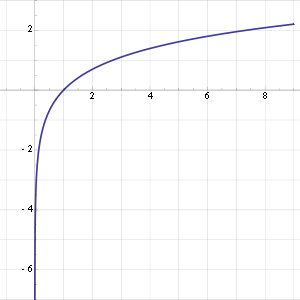Graph of the natural logarithm function. The function slowly grows to positive infinity as x increases and slowly goes to negative infinity as x approaches 0 ("slowly" as compared to any power law of x); the y-axis is an asymptote.

The natural logarithm of a number is its logarithm to the base of the mathematical constant e, where e is an irrational and transcendental number approximately equal to 2.718281828459. The natural logarithm of x is generally written as ln x, loge x, or sometimes, if the base e is implicit, simply log x. Parentheses are sometimes added for clarity, giving ln(x), loge(x) or log(x). This is done in particular when the argument to the logarithm is not a single symbol, to prevent ambiguity.

The natural logarithm of x is the power to which e would have to be raised to equal x. For example, ln(7.5) is 2.0149..., because e2.0149... = 7.5. The natural log of e itself, ln(e), is 1, because e1 = e, while the natural logarithm of 1, ln(1), is 0, since e0 = 1.

The natural logarithm can be defined for any positive real number a as the area under the curve y = 1/x from 1 to a (the area being taken as negative when a < 1). The simplicity of this definition, which is matched in many other formulas involving the natural logarithm, leads to the term "natural". The definition of the natural logarithm can be extended to give logarithm values for negative numbers and for all non-zero complex numbers, although this leads to a multi-valued function: see Complex logarithm.

The natural logarithm function, if considered as a real-valued function of a real variable, is the inverse function of the exponential function, leading to the identities:Like all logarithms, the natural logarithm maps multiplication into addition:Thus, the logarithm function is a group isomorphism from positive real numbers under multiplication to the group of real numbers under addition, represented as a function:Logarithms can be defined to any positive base other than 1, not only e. However, logarithms in other bases differ only by a constant multiplier from the natural logarithm, and are usually defined in terms of the latter. For instance, the binary logarithm is the natural logarithm divided by ln(2), the natural logarithm of 2. Logarithms are useful for solving equations in which the unknown appears as the exponent of some other quantity. For example, logarithms are used to solve for the half-life, decay constant, or unknown time in exponential decay problems. They are important in many branches of mathematics and the sciences and are used in finance to solve problems involving compound interest.

By Lindemann–Weierstrass theorem, the natural logarithm of any positive algebraic number other than 1 is a transcendental number.

 Natural logarithm RepresentationInverseDerivativeIndefinite Integral## History

Main article: History of logarithms

The concept of the natural logarithm was worked out by Gregoire de Saint-Vincent and Alphonse Antonio de Sarasa before 1649. Their work involved quadrature of the hyperbola xy = 1 by determination of the area of hyperbolic sectors. Their solution generated the requisite "hyperbolic logarithm" function having properties now associated with the natural logarithm.

An early mention of the natural logarithm was by Nicholas Mercator in his work Logarithmotechnia published in 1668, although the mathematics teacher John Speidell had already in 1619 compiled a table of what in fact were effectively natural logarithms.

## Notational conventions

The notations "ln x" and "loge x" both refer unambiguously to the natural logarithm of x. "log x" without an explicit base may also refer to the natural logarithm. This usage is common in mathematics and some scientific contexts as well as in many programming languages. In some other contexts, however, "log x" can be used to denote the common (base 10) logarithm.

Historically, the notations "l." and "l" were in use at least since the 1730s, and until at least the 1840s, then "log." or "log", at least since the 1790s. Finally, in the twentieth century, the notations "Log" and "logh" are attested.

## Origin of the term natural logarithm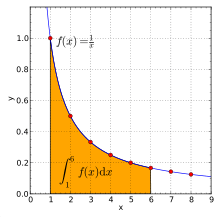The hyperbola y = 1/x (red curve) and the area from x = 1 to 6 (shaded in orange). This area equals the natural logarithm of 6.

The graph of the natural logarithm function shown earlier on the right side of the page enables one to glean some of the basic characteristics that logarithms to any base have in common. Chief among them are: the logarithm of the number one is zero; and the logarithm as x approaches zero from the right is negative infinity.

What makes natural logarithms unique is to be found at the single point where all logarithms are zero, namely the logarithm of the number one. At that specific point the "slope" of the curve of the graph of the natural logarithm is also precisely one. Logarithms to a higher base than e, such as those to the base 10, exhibit a slope at that point less than one, while logarithms to a lower base than e, such as those to the base 2, exhibit a slope at that point greater than one. While the methods for computing the "value" of e are fascinating from various mathematical perspectives, they all can be thought of as resulting from the pursuit of this condition.

Another way of conceptualizing this is to realize that, for any numeric value close to the number one, the natural logarithm can be computed by subtracting the number one from the numeric value. For example, the natural logarithm of 1.01 is 0.01 to an accuracy better than 5 parts per thousand. With similar accuracy one can assert that the natural logarithm of 0.99 is negative 0.01. The accuracy of this concept increases as one approaches the number one ever more closely, and reaches completeness of accuracy precisely there. To the same extent that the number one itself is a number common to all systems of counting, so also the natural logarithm is independent of all systems of counting. In the English language the term adopted to encapsulate this concept is the word "natural".

Initially, it might seem that since the common numbering system is base 10, this base would be more "natural" than base e. But mathematically, the number 10 is not particularly significant. Its use culturally—as the basis for many societies’ numbering systems—likely arises from humans’ typical number of fingers. Other cultures have based their counting systems on such choices as 5, 8, 12, 20, and 60.

loge is a "natural" log because it automatically springs from, and appears so often in, mathematics. For example, consider the problem of differentiating a logarithmic function:If the base b equals e, then the derivative is simply 1/x, and at x = 1 this derivative equals 1. Another sense in which the base-e-logarithm is the most natural is that it can be defined quite easily in terms of a simple integral or Taylor series and this is not true of other logarithms.

Further senses of this naturalness make no use of calculus. As an example, there are a number of simple series involving the natural logarithm. Pietro Mengoli and Nicholas Mercator called it logarithmus naturalis a few decades before Newton and Leibniz developed calculus.

## Definitions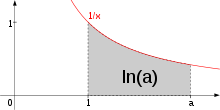ln(a) illustrated as the area under the curve f(x) = 1/x from 1 to a. If a is less than 1, the area from a to 1 is counted as negative.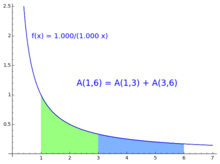The area under the hyperbola satisfies the logarithm rule. Here A(s,t) denotes the area under the hyperbola between s and t.

Formally, ln(a) may be defined as the area under the hyperbola 1/x. This is the integral,This function is a logarithm because it satisfies the fundamental property of a logarithm:This can be demonstrated by splitting the integral that defines ln(ab) into two parts and then making the variable substitution x = ta in the second part, as follows:In elementary terms, this is simply scaling by 1/a in the horizontal direction and by a in the vertical direction. Area does not change under this transformation, but the region between a and ab is reconfigured. Because the function a/(ax) is equal to the function 1/x, the resulting area is precisely ln(b).

The number e is defined as the unique real number a such that ln(a) = 1.

Alternatively, if the exponential function has been defined first, say by using an infinite series, the natural logarithm may be defined as its inverse function, i.e., ln is that function such that exp(ln(x)) = x. Since the range of the exponential function on real arguments is all positive real numbers and since the exponential function is strictly increasing, this is well-defined for all positive x.

## Properties

•••••••••## Derivative, Taylor series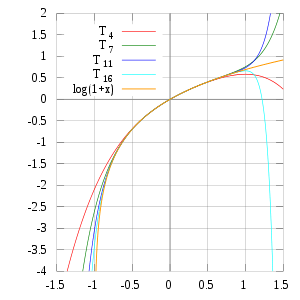The Taylor polynomials for ln(1 + x) only provide accurate approximations in the range −1 < x  1. Note that, for x > 1, the Taylor polynomials of higher degree are worse approximations.

The derivative of the natural logarithm is given byProof 1 by the first part of the fundamental theorem of calculus:Proof 2 from this video:This leads to the Taylor series for ln(1 + x) around 0; also known as the Mercator series(Leonhard Euler nevertheless boldly applied this series to x= -1, in order to show that the harmonic series equals the (natural) logarithm of 1/(1-1), that is the logarithm of infinity. Nowadays, more formally but perhaps less vividly, we prove that the harmonic series truncated at N is close to the logarithm of N, when N is large.)

At right is a picture of ln(1 + x) and some of its Taylor polynomials around 0. These approximations converge to the function only in the region −1 < x  1; outside of this region the higher-degree Taylor polynomials are worse approximations for the function.

Substituting x  1 for x, we obtain an alternative form for ln(x) itself, namelyBy using the Euler transform on the Mercator series, one obtains the following, which is valid for any x with absolute value greater than 1:This series is similar to a BBP-type formula.

Also note thatis its own inverse function, so to yield the natural logarithm of a certain number y, simply put infor x.## The natural logarithm in integration

The natural logarithm allows simple integration of functions of the form g(x) = f '(x)/f(x): an antiderivative of g(x) is given by ln(|f(x)|). This is the case because of the chain rule and the following fact:In other words,andHere is an example in the case of g(x) = tan(x):Letting f(x) = cos(x) and f'(x)= – sin(x):where C is an arbitrary constant of integration.

The natural logarithm can be integrated using integration by parts:Let:then:## Numerical value

To calculate the numerical value of the natural logarithm of a number, the Taylor series expansion can be rewritten as:To obtain a better rate of convergence, the following identity can be used.provided that y = (x−1)/(x+1) and Re(x) > 0.

For ln(x) where x > 1, the closer the value of x is to 1, the faster the rate of convergence. The identities associated with the logarithm can be leveraged to exploit this:Such techniques were used before calculators, by referring to numerical tables and performing manipulations such as those above.

### Natural logarithm of 10

The natural logarithm of 10, which has the decimal expansion 2.30258509..., plays a role for example in the computation of natural logarithms of numbers represented in scientific notation, as a mantissa multiplied by a power of 10:This means that one can effectively calculate the logarithms of numbers with very large or very small magnitude using the logarithms of a relatively small set of decimals in the range.

### High precision

To compute the natural logarithm with many digits of precision, the Taylor series approach is not efficient since the convergence is slow. If x is near 1, an alternative is to use Newton's method to invert the exponential function, whose series converges more quickly. For an optimal function, the iteration simplifies towhich has cubic convergence to ln(x).

Another alternative for extremely high precision calculation is the formulawhere M denotes the arithmetic-geometric mean of 1 and 4/s, andwith m chosen so that p bits of precision is attained. (For most purposes, the value of 8 for m is sufficient.) In fact, if this method is used, Newton inversion of the natural logarithm may conversely be used to calculate the exponential function efficiently. (The constants ln 2 and π can be pre-computed to the desired precision using any of several known quickly converging series.)

Based on a proposal by William Kahan and first implemented in the Hewlett-Packard HP-41C calculator in 1979, some calculators, computer algebra systems and programming languages (for example C99) provide a special natural logarithm plus 1 function alternatively named LN1+X, LN(1+X), lnp1(x), ln1p(x) or log1p(x) to give more accurate results for values of x close to zero compared to using ln(x+1) directly. This function is implemented using a different internal algorithm to avoid an intermediate result near 1, thereby allowing both the argument and the result to be near zero. Similar inverse functions named expm1(x), expm(x) or exp1m(x) exist as well.[nb 1]

### Computational complexity

The computational complexity of computing the natural logarithm (using the arithmetic-geometric mean) is O(M(n) ln n). Here n is the number of digits of precision at which the natural logarithm is to be evaluated and M(n) is the computational complexity of multiplying two n-digit numbers.

## Continued fractions

While no simple continued fractions are available, several generalized continued fractions are, including:These continued fractions—particularly the last—converge rapidly for values close to 1. However, the natural logarithms of much larger numbers can easily be computed by repeatedly adding those of smaller numbers, with similarly rapid convergence.

For example, since 2 = 1.253 × 1.024, the natural logarithm of 2 can be computed as:Furthermore, since 10 = 1.2510 × 1.0243, even the natural logarithm of 10 similarly can be computed as:## Complex logarithms

Main article: Complex logarithm

The exponential function can be extended to a function which gives a complex number as ex for any arbitrary complex number x; simply use the infinite series with x complex. This exponential function can be inverted to form a complex logarithm that exhibits most of the properties of the ordinary logarithm. There are two difficulties involved: no x has ex = 0; and it turns out that e2πi = 1 = e0. Since the multiplicative property still works for the complex exponential function, ez = ez+2πki, for all complex z and integers k.

So the logarithm cannot be defined for the whole complex plane, and even then it is multi-valued – any complex logarithm can be changed into an "equivalent" logarithm by adding any integer multiple of 2πi at will. The complex logarithm can only be single-valued on the cut plane. For example, ln(i) = πi/2 or 5πi/2 or -3πi/2, etc.; and although i4 = 1, 4 log(i) can be defined as 2πi, or 10πi or −6πi, and so on.

## Notes

1. For a similar approach to reduce round-off errors of calculations for certain input values see trigonometric functions like versine, vercosine, coversine, covercosine, haversine, havercosine, hacoversine, hacovercosine, exsecant and excosecant.

## References

1. Mortimer, Robert G. (2005). Mathematics for physical chemistry (3rd ed.). Academic Press. p. 9. ISBN 0-12-508347-5. Extract of page 9
2. R. P. Burn (2001) "Alphonse Antonio de Sarasa and Logarithms", Historia Mathematica 28:1 – 17
3. J J O'Connor and E F Robertson (September 2001). "The number e". The MacTutor History of Mathematics archive. Retrieved 2009-02-02.
4. Cajori, Florian (1991). A History of Mathematics, 5th ed. AMS Bookstore. p. 152. ISBN 0-8218-2102-4.
5. Including C, C++, SAS, MATLAB, Mathematica, Fortran, and BASIC
6. Euler, Leonhard (1737). "Variae observationes circa series inﬁnitas". Commentarii academiae scientiarum imperialis Petropolitanae (CASP) (published 1744). 9: 160–188. E72.
7. Euler, Leonhard (1925). Opera Omnia, Series Prima: Opera Mathematica. Quartum Decimum. Teubner.
8. Cauchy, Augustin. Exercices d'analyse et de physique mathématique. 3. p. 380. Retrieved 2015-10-31.
9. Legendre, Adrien-Marie (VI (1797 or 1798)). Essai sur la théorie des nombres. Paris, France: Duprat, libraire pour les mathématiques, quai des Augustins. Check date values in: |date= (help)
10. Landau, Edmund (1953) . Handbuch der Lehre von der Verteilung der Primzahlen (2 ed.). Berlin: Chelsea, New York.
11. Piskounov, Nikolaï (1972). Calcul différentiel et intégral, (5 ed.). Moskow: Editions Mir. p. 91.
12. Jolley, L.B.W. (1961). Summation of Series (PDF) (2 (revised) ed.). New York, USA: Dover Publications, Inc. LCCN 61-65274. Retrieved 2015-10-31.
13. Boyer, Carl Benjamin; Merzbach, Uta C. (1991-03-06) . A History of Mathematics (2 ed.). New York, USA: John Wiley & Sons. ISBN 978-0471543978. 0471543977.
14. Harris, John (1987). "Australian Aboriginal and Islander mathematics" (PDF). Australian Aboriginal Studies. 2: 29–37. Retrieved 2008-02-12.
15. Large, J.J. (1902). "The vigesimal system of enumeration". Journal of the Polynesian Society. 11 (4): 260–261. Retrieved 30 March 2011.
16. Cajori, Florian (1922). "Sexagesimal fractions among the Babylonians". American Mathematical Monthly. 29 (1): 8–10. doi:10.2307/2972914. JSTOR 2972914.
17. Larson, Ron (2007). Calculus: An Applied Approach (8th ed.). Cengage Learning. p. 331. ISBN 0-618-95825-8., Section 4.5, page 331
18. Ballew, Pat. "Math Words, and Some Other Words, of Interest". Retrieved 2007-09-16.
19. Leonhard Euler, Introductio in Analysin Infinitorum. Tomus Primus. Bousquet, Lausanne 1748. Exemplum 1, p. 228; quoque in: Opera Omnia, Series Prima, Opera Mathematica, Volumen Octavum, Teubner 1922
20. "Logarithmic Expansions" at Math2.org
21. Sasaki, T.; Kanada, Y. (1982). "Practically fast multiple-precision evaluation of log(x)". Journal of Information Processing. 5 (4): 247–250. Retrieved 30 March 2011.
22. Ahrendt, Timm (1999). "Stacs 99". Lecture notes in computer science. 1564: 302–312. doi:10.1007/3-540-49116-3_28. ISBN 978-3-540-65691-3. |chapter= ignored (help)
23. Beebe, Nelson H. F. (2002-07-09). "Computation of expm1 = exp(x)−1" (PDF). 1.00. Salt Lake City, Utah, USA: Department of Mathematics, Center for Scientific Computing, University of Utah. Retrieved 2015-11-02.
24. HP 48G Series – Advanced User's Reference Manual (AUR) (4 ed.). Hewlett-Packard. December 1994 . HP 00048-90136, 0-88698-01574-2. Retrieved 2015-09-06.
25. HP 50g / 49g+ / 48gII graphing calculator advanced user’s reference manual (AUR) (2 ed.). Hewlett-Packard. 2009-07-14 . HP F2228-90010. Retrieved 2015-10-10.Searchable PDF